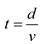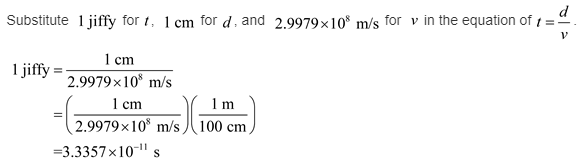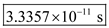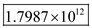# A Jiffy The American physical chemist Gilbert Newton Lewis (1875–1946) proposed a unit of time called the “jiffy

A Jiffy The American physical chemist Gilbert Newton Lewis (1875–1946) proposed a unit of time called the “jiffy.”According to Lewis, 1 jiffy = the time it takes light to travel one centimeter, (a) If you perform a task in a jiffy, how long has it taken in seconds? (b) How many jiffya are in one minute? (Use the fact that the speed of light is approximately 2.9979 x 108 m/s.)

CONCEPT:
The new unit proposed for time by the physical chemist is called as “jiffy”. It is equal to the time taken by light to travel a distance of 1 cm.
The relation for time taken in terms of distance and speed isHere, d is distance and v is speed.

SOLUTION:
(a) The time taken by the task is measured in seconds as follows.
Convert the unit jiffy into seconds.Therefore, the time taken by the task is measured in seconds is(b) The number of jiffys in minute is calculated as follows.Therefore, the number of jiffys in minute is